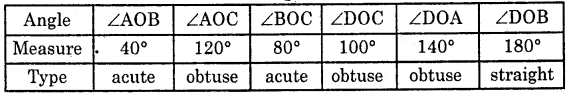# HBSE 6th Class Maths Solutions Chapter 5 Understanding Elementary Shapes Ex 5.4

Haryana State Board HBSE 6th Class Maths Solutions Chapter 5 Understanding Elementary Shapes Ex 5.4 Textbook Exercise Questions and Answers.

## Haryana Board 6th Class Maths Solutions Chapter 5 Understanding Elementary Shapes Exercise 5.4

Question 1.
What is the measure of (i) a right angle, (ii) a straight angle ?
Solution:
(i) The measure of a right angle is 90°.
(ii) The measure of a straight angle is 180°.Question 2.
Say True or False :
(a) The measure of an acute angle < 90°.
(b) The measure of an obtuse angle < 90°.
(c) The measure of a reflex angle > 180°.
(d) The measure of one complete revolution = 360°.
(e) If m ∠A = 530 and m ∠B 35°, then m∠A > m ∠B.
Solution:
(a) True,
(b) False,
(c) True,
(d) True,
(e) True.Question 3.
Write down the measures of:
(a) some acute angles.
(b) some obtuse angles.
(Give two examples of each).
Solution:
(a) 30° and 60° are acute angles.
(b) 110° and 120° are obtuse angles.

Question 4.
Measure the angles given below, using the Protractor and write down the measure.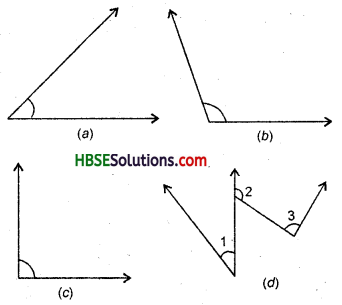Solution:
(a) 45°,
(b) 125°,
(c) 90°,
(d) ∠1 = 40°, ∠2 = 125°, ∠3 = 95°.Question 5.
Which angle has a large measure ?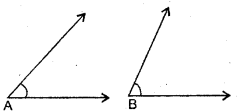First estimate and then measure. Measure of Angle A =
Measure of Angle B =
Solution:
Measure of ∠A = 40° and measure of ∠B = 65°
∴ ∠B > ∠A.

Question 6.
From these two angles, which has a larger measure ? Estimate and then confirm by measuring them.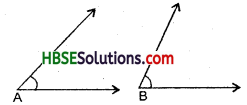Solution:
∠A = 45° and ∠B = 60°
∴ ∠B > ∠A.

Question 7.
Fill in the blanks with acute, obtuse, right or straight :
(a) An angle whose measure is less than that of a right angle is ………………
(b) An angle whose measure is greater than that of a right angle is ………………
(c) An angle whose measure is the sum of the measures of two right angles is ………………
(d) When the sum of the measures of two angles is that of a right angle, then each one of them is ………………
(e) When the sum of the measures of two angles is that of a straight angle, one of them should be ……………… or ………………
Solution:
(a) acute,
(b) obtuse,
(c) straight,
(d) acute,
(e) acute, obtuse.

Question 8.
Find the measure of the angle shown in each figure. (First estimate with your eye and then find the actual measure with a protractor).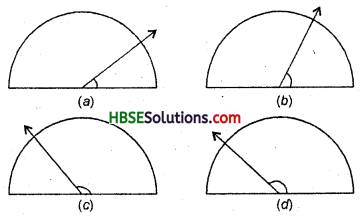Solution:
(a) 40°, (b) 65°, (c) 130°, (d) 135°.Question 9.
Find the angle measure between the hands of the clock in each figure :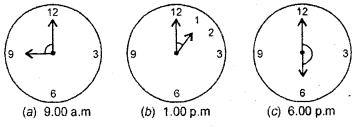Solution:
(a) 90°, (b) 30°, (d) 180°.

Question 10.
Investigate : In the given figures, protractor shows 30° Look at the same figure through a magnifying glass. Does the angle becomes larger ? Does the size of the angle change ?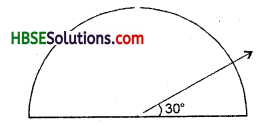Solution:
No, No.

Question 11.
Measure and classify each angle.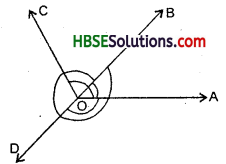Solution: